# Parallel in Parallel Out (PIPO) Shift Register

Parallel In Parallel Out (PIPO) shift registers are the type of storage devices in which both data loading as well as data retrieval processes occur in parallel mode. Figure 1 shows a PIPO register capable of storing n-bit input data word (Data in). Here each flip-flop stores an individual bit of the data in appearing as its input (FF1 stores B1 appearing at D1; FF2 stores B2 appearing at D2 … FFn stores Bn appearing at Dn) at the instant of first clock pulse. Further, at the same instant, the bit stored in each individual flip-flop also appears at their respective output pins (Q1 = D1; Q2 = D2 … Qn = Bn). This indicates that both data storage as well as data recovery occur at a single (and at the same) clock pulse in PIPO registers.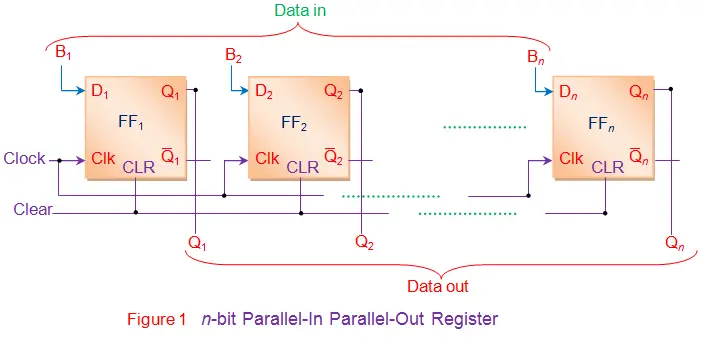However one has to note that the PIPO register shown in Figure 1 is not capable of shifting the data bits. In order to convert PIPO register of Figure 1 into PIPO shift register, one has to modify its circuit by adding combinational circuit and control line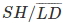as shown by Figure 2.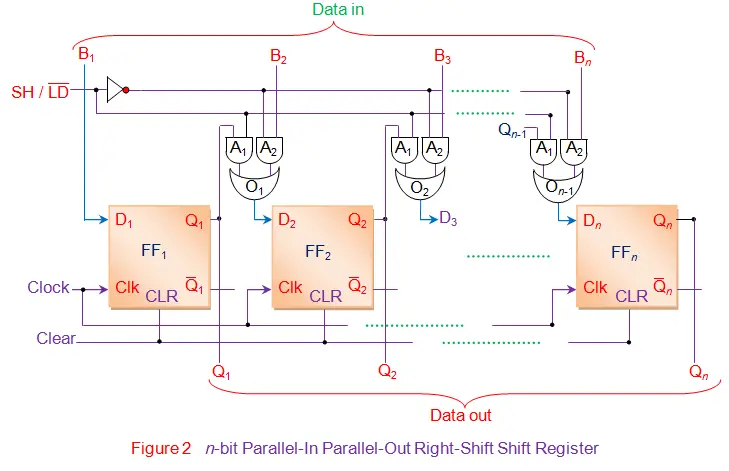Here ifline goes low, A2 AND gates of all the combinational circuits become active while A1 gates become inactive.
Thus the bits of the input data word (Data in) appearing as inputs to the gates A2 are passed on as the OR gate outputs which are further loaded/stored into respective flip-flops at the appearance of first leading edge of the clock (except the bit B1 which gets directly stored into FF1 at the first clock tick). This indicates that all the bits of the input data word are stored into the register components at the same clock tick. At the same time, these bits also appear at the output pins of the respective flip-flops thus yielding parallel-output data word at the same clock tick.

Further whenline is made high, A1 gates of all the combinational circuits enable while A2 gates get disabled. This causes the output bit of each flip-flop to appear at the output of the OR gate driving the very-next flip-flop (except the last flip-flop FFn) i.e. output bit of FF1 (Q1) appears as the output of OR gate 1 (O1) connected to D2; Q2 = output of O2 = D3 and so on. At this stage, if the rising edge of the clock pulse appears, then Q1 appears at Q2, Q2 appears at Q3, … and Qn-1 appears at Qn. This is nothing but right-shift of the data stored within the register by one-bit. This working is further emphasized in the Table I and Figure 3.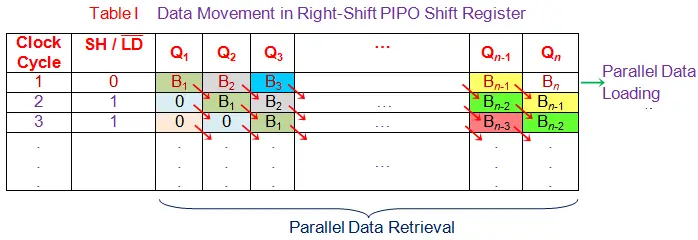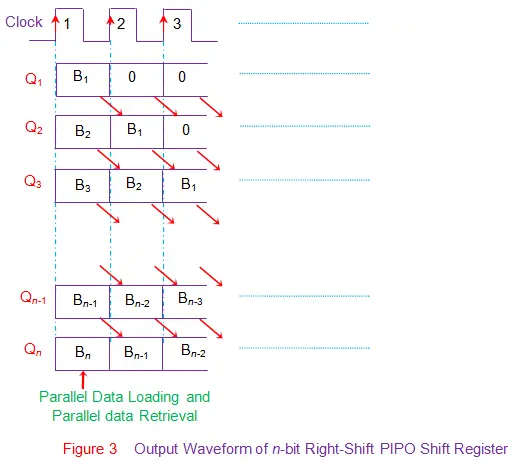Similar to the right-shift PIPO shift register, there can also be a left-shift PIPO shift register as shown by Figure 4. Nevertheless the mode of working remains the same.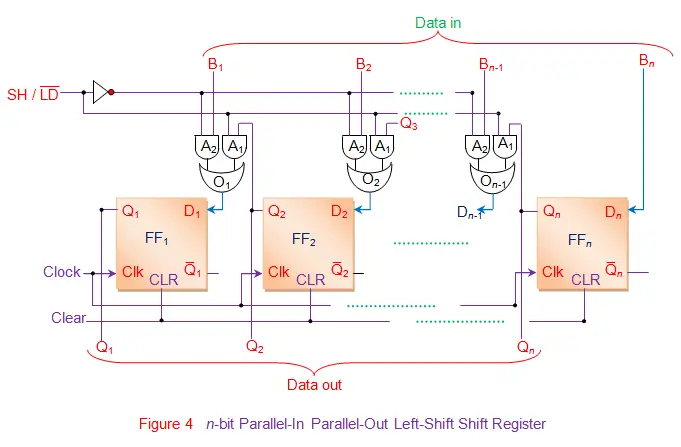Want To Learn Faster? 🎓
Get electrical articles delivered to your inbox every week.
No credit card required—it’s 100% free.